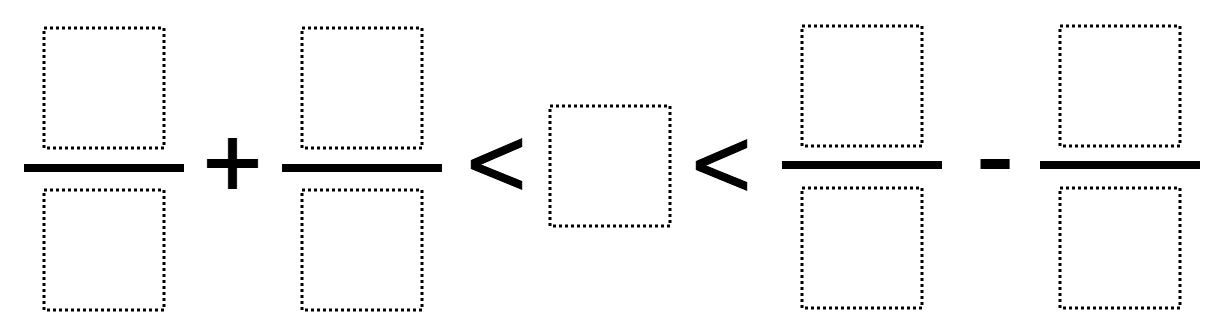Home > Grade 7 > Expressions & Equations > Inequality Expressions 3

# Inequality Expressions 3

Directions: Using the digits 1 to 9 at most one time each, fill in the boxes to create a true inequality.### Hint

What happens when you add or subtract Rational Numbers?

### Answer

There are multiple correct answers, one example would be (4/8) + (6/7) < 5 < (9/1) – (2/3)

Source: Bryan Anderson

## Converting a Fraction to a Decimal

Directions: Using the digits 1 to 9, at most one time each, place a digit …